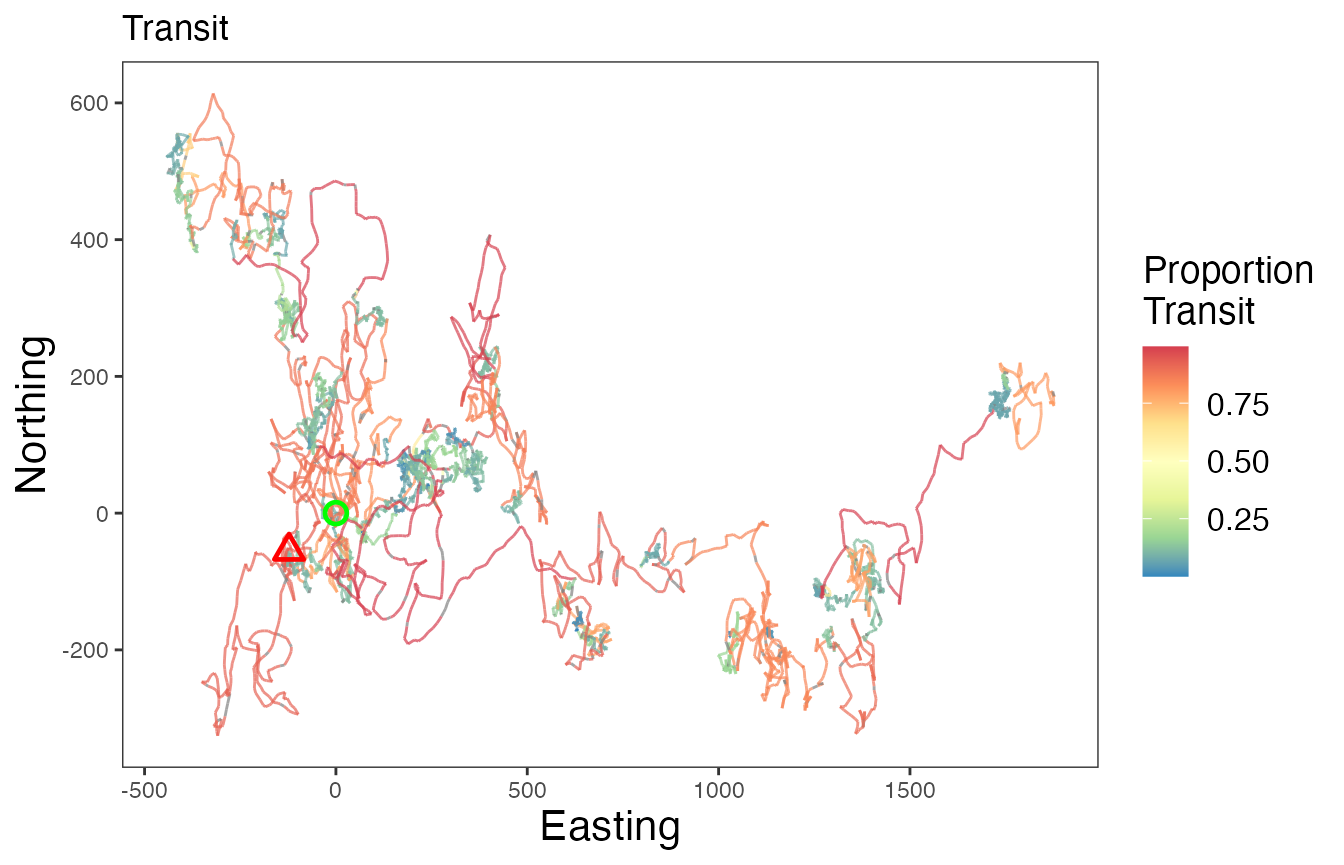## Introduction

There may be instances where the dataset of interest has structure that should be accounted for by the model.

For example, devices such as Argos satellite tags on air-breathing marine megafauna may have a variable denoting that the animal has “hauled-out”, meaning that they’re on land. This is particularly common for different seal species, as well as nesting sea turtles. In a similar vein, terrestrial some terrestrial species may go into underground burrows each day to rest, which may be denoted by the loss of a GPS signal until the animal re-emerges. Researchers may also just be interested in the diel patterns of movement exhibited by many species.

### Pre-specification of breakpoints

Each of these examples demonstrates a priori knowledge about the species of interest or the inclusion of a variable denoting a specific behavior can be associated with a particular behavior. This knowledge can be directly leveraged by the model that segments the tracks and then clusters them into behavioral states by allowing users to pre-specify breakpoints. These pre-specified breakpoints are then supplied when running the segmentation model and the reversible-jump Markov chain Monte Carlo algorithm considers whether to keep them, shift them over, or delete them altogether. In some cases, this can dramatically speed up time to convergence for the Bayesian model. It is worth noting that the model may remove nearly all of the pre-specified breakpoints. In these instances, a number of things may be going wrong: 1) there are too many missing values (NAs) in the data streams, 2) the bins do not characterize the data streams well, and 3) the method or variable used to define the pre-specified breakpoints does not identify any underlying structure in the data streams being analyzed.

### Handling of zero-inflated data streams

Another characteristic of some data streams included for analysis are zero-inflated data streams. This means that certain variables have a high number of zeroes with respect to other real numbers or integers. The segmentation-clustering model as well as the clustering-only model that estimates behavioral states at the observation-level can easily account for this structure in the data by lumping all zeroes of a given variable into a single bin of the discretized data stream. This also makes interpretation of the state-dependent distributions quite easy when interpreting each of the different estimated behavioral states.

### Handling of missing values

While unrelated to the above topics, it is also worth mentioning that both state estimation models (segment-level or observation-level behavioral states) can handle NAs. This requires no. While there are no specific guidelines on the proportion of NA values that can be included for one or more data streams, those with greater numbers of NAs will hold less weight in influencing the estimation of breakpoints compared to those will full data streams.

A demonstration will be provided below on analyzing a dataset with a “known” set of possible breakpoints as well as the analysis of a zero-inflated variable using the built in tracks dataset in the bayesmove package.

## Prepare data

This tutorial works under the assumption that readers have covered the previous vignettes for the segmentation-level state estimation model since there won’t be much description on many of the steps repeated here. First, let’s load in the data and visualize some of its attributes.

library(bayesmove)
library(tidyverse)
library(future)

data(tracks)

# Check data structure
#>    id                date        x          y
#> 1 id1 2020-07-02 11:59:41  0.00000  0.0000000
#> 2 id1 2020-07-02 12:58:26 10.56492 -1.6654990
#> 3 id1 2020-07-02 13:59:31 25.50174 -0.6096675
#> 4 id1 2020-07-02 15:01:27 31.22014  9.5438464
#> 5 id1 2020-07-02 15:59:56 36.15821 19.8737009
#> 6 id1 2020-07-02 16:58:38 39.06810 26.4996352
unique(tracks$id) #>  "id1" "id2" "id3"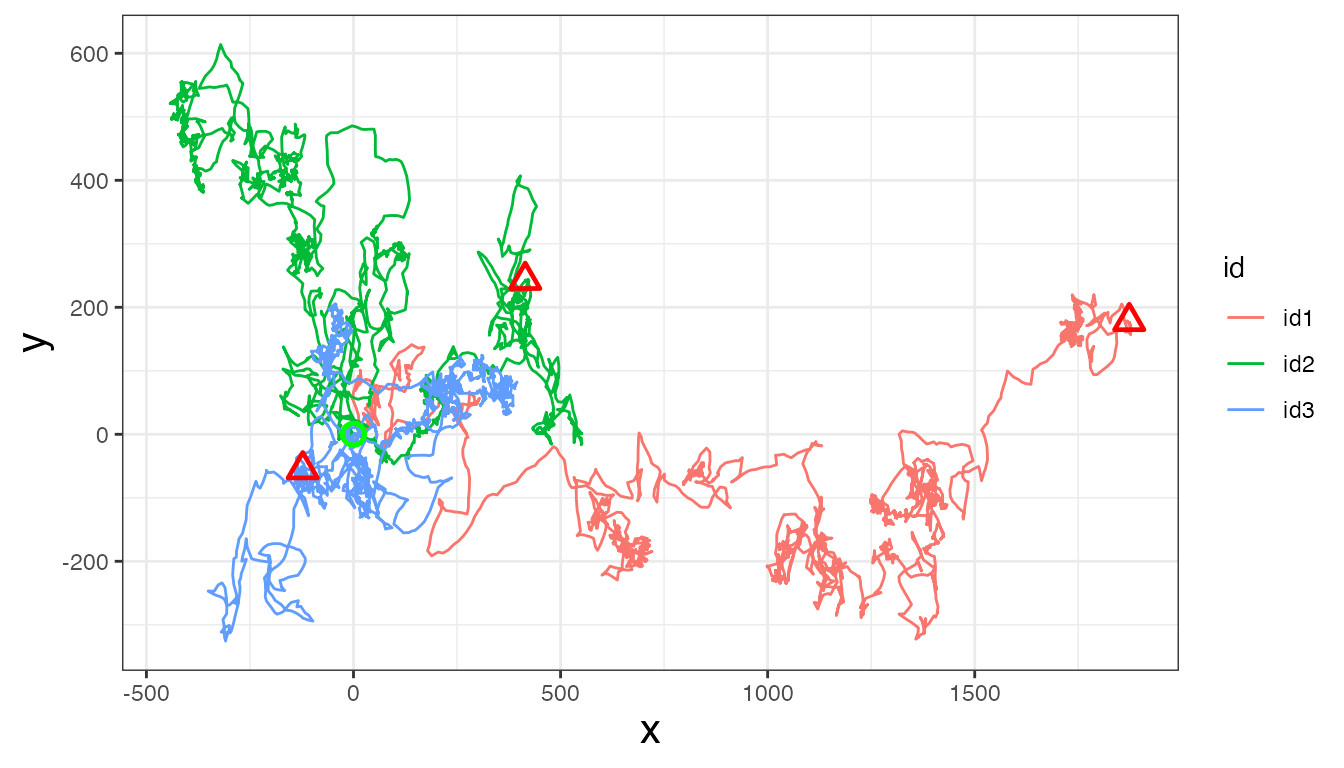Now, let’s calculate step length, turning angle, net-squared displacement, and time step: tracks<- prep_data(dat = tracks, coord.names = c("x","y"), id = "id") head(tracks) #> id date x y step angle NSD dt #> 1 id1 2020-07-02 11:59:41 0.00000 0.0000000 10.695 NA 0.000 3526 #> 2 id1 2020-07-02 12:58:26 10.56492 -1.6654990 14.974 0.227 114.392 3664 #> 3 id1 2020-07-02 13:59:31 25.50174 -0.6096675 11.653 0.987 650.710 3716 #> 4 id1 2020-07-02 15:01:27 31.22014 9.5438464 11.449 0.067 1065.782 3509 #> 5 id1 2020-07-02 15:59:56 36.15821 19.8737009 7.237 0.032 1702.380 3522 #> 6 id1 2020-07-02 16:58:38 39.06810 26.4996352 0.119 -2.804 2228.547 3738 Next, we will round time steps (and dates) to a specified interval (1 h; 3600 s) if within 3 minutes (180 s) of this interval. tracks<- round_track_time(dat = tracks, id = "id", int = 3600, tol = 180, time.zone = "UTC", units = "secs") head(tracks) #> id date x y step angle NSD dt #> 1 id1 2020-07-02 11:59:41 0.00000 0.0000000 10.695 NA 0.000 3600 #> 2 id1 2020-07-02 12:59:41 10.56492 -1.6654990 14.974 0.227 114.392 3600 #> 3 id1 2020-07-02 13:59:41 25.50174 -0.6096675 11.653 0.987 650.710 3600 #> 4 id1 2020-07-02 14:59:41 31.22014 9.5438464 11.449 0.067 1065.782 3600 #> 5 id1 2020-07-02 15:59:41 36.15821 19.8737009 7.237 0.032 1702.380 3600 #> 6 id1 2020-07-02 16:59:41 39.06810 26.4996352 0.119 -2.804 2228.547 3600 ### Artificially define ‘resting’ behavior To demonstrate how to pre-specify breakpoints, I will be creating a synthetic variable that denotes a ‘resting’ behavioral state based on the step length variable. A step like this one in the creation of a derived variable may or may not be necessary for your particular dataset. In this case, I will find the lower quartile of step lengths and use this as the threshold to define the ‘resting’ behavior. quantile(tracks$step, 0.25, na.rm = T)
#>   25%
#> 0.106

Across all three tracks, the lower quartile is almost exactly 0.1, so this will be the value used as the threshold for a resting vs not resting state. Additionally, I will change all step length values below this threshold to zero in order to artificially increase the zeroes in this datastream. The following code demonstrates how I do this and create the new binary variable denoting resting from non-resting observations. Since each of the state estimation models requires the analysis of discretized variables denoted by positive integers, I will create a binary rest variable that is denoted by 1 (“rest”) and 2 (“non-rest”) rather than 0 and 1.

tracks <- tracks %>%
mutate(step = case_when(step <= 0.1 ~ 0,
step > 0.1 ~ step)
) %>%
mutate(rest = case_when(step > 0.1 ~ 2,
step == 0 ~ 1)
)

Next, the data need to be reformatted as a list where each element is a different individual track. As a list object, the data will then be filtered to only retain observations at a 1 hr (3600 s) time interval.

# Create list from data frame
tracks.list<- df_to_list(dat = tracks, ind = "id")

# Filter observations
tracks_filt.list<- filter_time(dat.list = tracks.list, int = 3600)

### Discretize data streams

Before the data streams can be analyzed by the model, they must first be discretized into bins. Further details are provided in the Prepare data for analysis and Cluster observations vignettes. Since the ‘rest’ variable is binary and has already been coded as a 1 or 2, it is already technically discretized. So the remainder of the work needs to be done on the other two data streams included in this analysis, the step lengths and turning angles.

# Define bin number and limits for turning angles
angle.bin.lims=seq(from=-pi, to=pi, by=pi/4)  #8 bins

# Define bin number and limits for step lengths
dist.bin.lims=quantile(tracks[tracks$dt == 3600,]$step,
c(0,0.25,0.50,0.75,0.90,1), na.rm=T)  #5 bins

angle.bin.lims
#>  -3.1415927 -2.3561945 -1.5707963 -0.7853982  0.0000000  0.7853982  1.5707963
#>   2.3561945  3.1415927

dist.bin.lims
#>       0%      25%      50%      75%      90%     100%
#>  0.00000  0.10700  1.27900  5.73825 10.75250 25.25200

# Assign bins to observations
tracks_disc.list<- map(tracks_filt.list,
discrete_move_var,
lims = list(dist.bin.lims, angle.bin.lims),
varIn = c("step", "angle"),
varOut = c("SL", "TA"))

Now that we have two new columns in the dataset representing the discretized step lengths (SL) and turning angles (TA), we need to create a 6th bin for step lengths to hold all of the zeroes. This is because they are currently all stored within the first bin with other non-zero values.

# Since 0s get lumped into bin 1 for SL, need to add a 6th bin to store only 0s
tracks_disc.list2 <- tracks_disc.list %>%
map(., ~mutate(.x, SL = SL + 1)) %>%  #to shift bins over from 1-5 to 2-6
map(., ~mutate(.x, SL = case_when(step == 0 ~ 1,  #assign 0s to bin 1
step != 0 ~ SL)  #otherwise keep the modified SL bin
))

## Segment the tracks

With the newly discretized data streams, the tracks can now be analyzed by the segmentation model. However, we still have yet to pre-sepcify the breakpoints.

### Pre-specify breakpoints

To pre-specify breakpoints, users will apply the find_breaks() function. In its standard form, this function can only be applied to one track at a time, so we will use purrr::map() to map this function across each of the list elements (i.e. different tracks). We also need to supply a character vector to the argument ind, which indicates the column name on which we would like to identify possible breakpoints. This function only works on discrete variables (i.e., integers), so users will need to potentially modify the variable supplied or modify this function to meet the needs of their data. If pre-specifying breakpoints for dates to denote diel patterns, users could potentially apply this function to the day-of-year or Julian day.

Since the pre-specified breakpoints must be supplied to the model as a list, the resulting breaks object shows how these potential breakpoints should be stored.

# Only retain id, discretized step length (SL), turning angle (TA), and rest columns
tracks.list2<- map(tracks_disc.list2,
subset,
select = c(id, SL, TA, rest))

# Pre-specify breakpoints based on 'rest'
breaks<- map(tracks.list2, ~find_breaks(dat = ., ind = "rest"))

### Run the segmentation model

set.seed(1)

# Define hyperparameter for prior distribution
alpha<- 1

# Set number of iterations for the Gibbs sampler
ngibbs<- 50000

# Set the number of bins used to discretize each data stream
nbins<- c(6,8,2)  #SL, TA, rest (in the order from left to right in tracks.list2)

progressr::handlers(progressr::handler_progress(clear = FALSE))
future::plan(future::multisession, workers = 3)  #run all MCMC chains in parallel
#refer to future::plan() for more details

dat.res<- segment_behavior(data = tracks.list2, ngibbs = ngibbs, nbins = nbins,
alpha = alpha, breakpt = breaks)
#> 103.66 sec elapsed
# takes 1.5 min to run

future::plan(future::sequential)  #return to single core

Before we proceed further with these estimated breakpoints from the model, let’s check that it has converged first by inspecting traceplots of the log marginal likelihood and the number of breakpoints.

# Trace-plots for the number of breakpoints per ID
traceplot(data = dat.res, type = "nbrks")# Trace-plots for the log marginal likelihood (LML) per ID
traceplot(data = dat.res, type = "LML")#both plots appear to indicate convergence to posterior

Everything looks good from the traceplots, so now let’s identify the maximum a posteriori (MAP) estimate that we’ll use in the next step.

## Determine MAP for selecting breakpoints
MAP.est<- get_MAP(dat = dat.res$LML, nburn = 25000) MAP.est #>  32049 34861 26213 brkpts<- get_breakpts(dat = dat.res$brkpts, MAP.est = MAP.est)

# How many breakpoints estimated per ID?
#> id1 id2 id3
#>  37  45  40

Another good visual diagnostic to check how well the model is able to identify major breakpoints consistent with a user’s a priori knowledge is to plot the breakpoints over the analyzed data streams.

plot_breakpoints(data = tracks_disc.list2, as_date = FALSE, var_names = c("step","angle","rest"),
var_labels = c("Step Length (km)", "Turning Angle (rad)", "Resting"), brkpts = brkpts)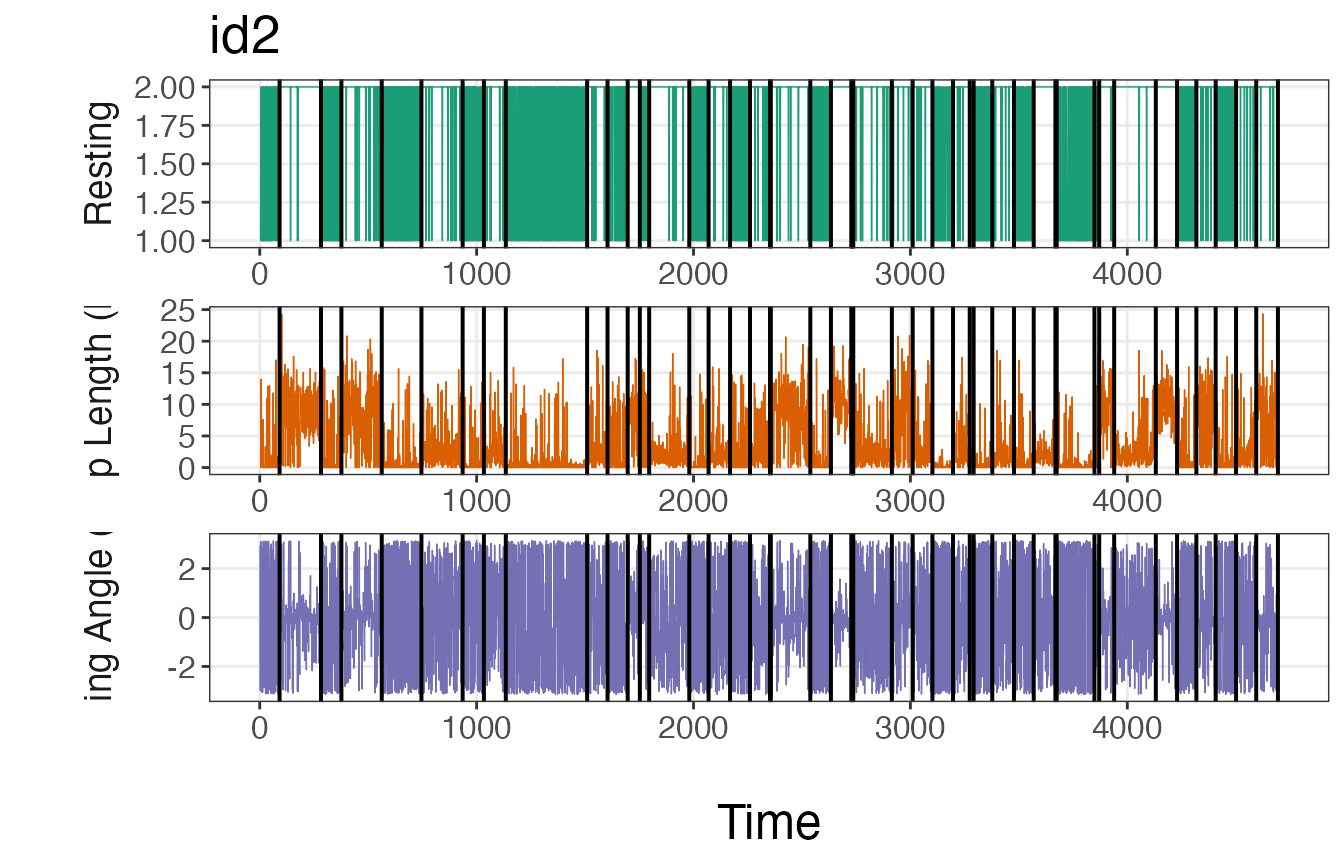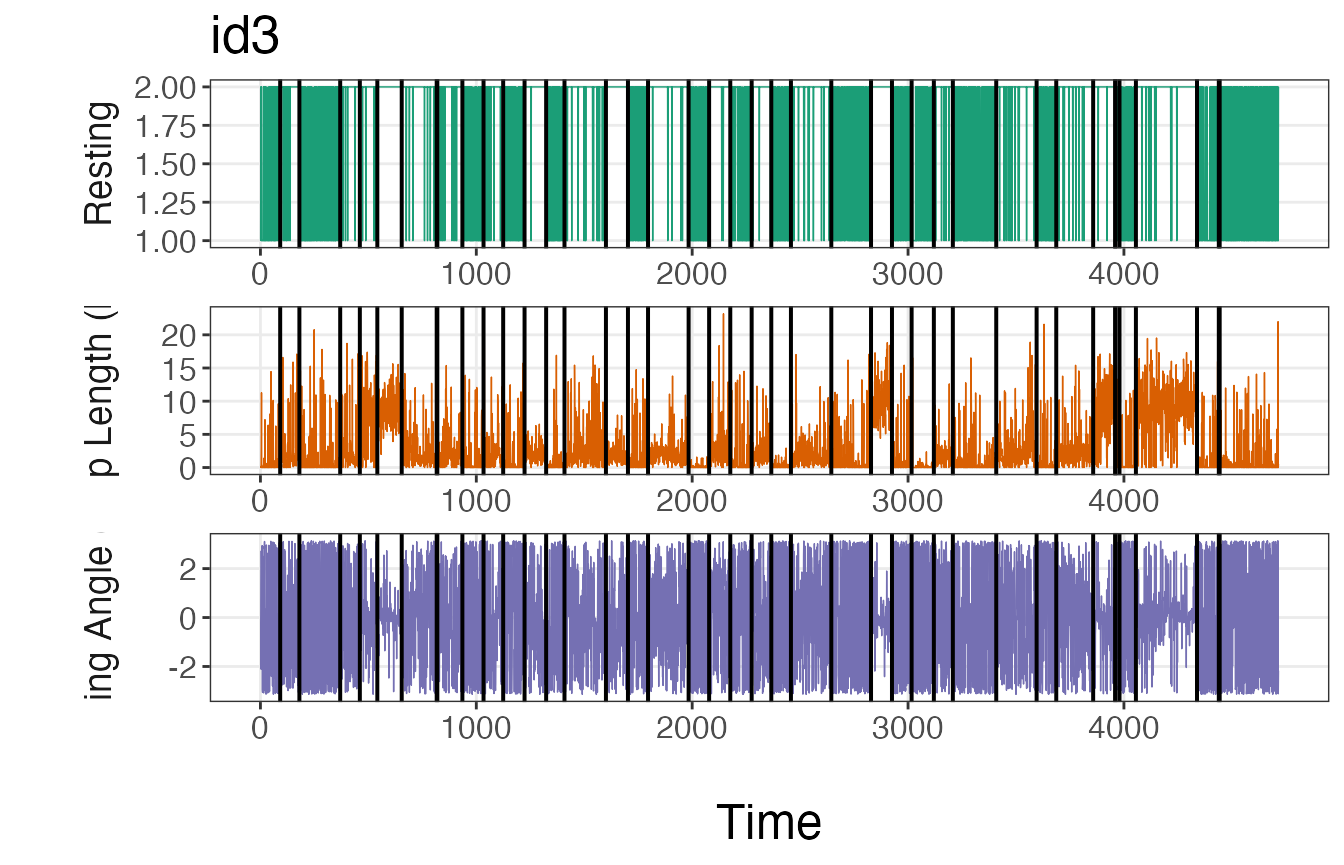If these plots match up with intuition, then it is safe to move forward with the estimated breakpoints. If the number or location of these breakpoints deviate from expectations, it may be necessary to run the model again with a greater number of iterations or using a different method to discretize the data streams.

### Assign track segments to tracks

With the modeled breakpoints, users will need to assign track segment numbers to each individual. This is performed using the assign_tseg() function.

tracks.seg<- assign_tseg(dat = tracks_disc.list2, brkpts = brkpts)

#>    id                date        x          y   step  angle      NSD   dt rest
#> 1 id1 2020-07-02 11:59:41  0.00000  0.0000000 10.695     NA    0.000 3600    2
#> 2 id1 2020-07-02 12:59:41 10.56492 -1.6654990 14.974  0.227  114.392 3600    2
#> 3 id1 2020-07-02 13:59:41 25.50174 -0.6096675 11.653  0.987  650.710 3600    2
#> 4 id1 2020-07-02 14:59:41 31.22014  9.5438464 11.449  0.067 1065.782 3600    2
#> 5 id1 2020-07-02 15:59:41 36.15821 19.8737009  7.237  0.032 1702.380 3600    2
#> 6 id1 2020-07-02 16:59:41 39.06810 26.4996352  0.119 -2.804 2228.547 3600    2
#>   obs time1 SL TA tseg
#> 1   1     1  5 NA    1
#> 2   2     2  6  5    1
#> 3   3     3  6  6    1
#> 4   4     4  6  5    1
#> 5   5     5  5  5    1
#> 6   6     6  3  1    1

## Cluster the segments

With track segments now denoted from the segmentation model, the next step is to cluster these segments together into behavioral states using Latent Dirichlet Allocation (LDA) using the cluster_segments() function. But first, we need to count the number of observations per segment that belong in each of the discretized bins.

# Select only id, tseg, SL, TA, and rest columns
tracks.seg2<- tracks.seg[,c("id","tseg","SL","TA","rest")]

# Summarize observations by track segment
nbins<- c(6,8,2)
obs<- summarize_tsegs(dat = tracks.seg2, nbins = nbins)
#>    id tseg y1.1 y1.2 y1.3 y1.4 y1.5 y1.6 y2.1 y2.2 y2.3 y2.4 y2.5 y2.6 y2.7
#> 1 id1    1    4    0    7   13   44   24    7    4    1   26   38    7    0
#> 2 id1    2   53    0   24    8    6    1   40    7    3    3    3    5    5
#> 3 id1    3    9    0    6   10   34   27    5    5    4   39   22    4    1
#> 4 id1    4    0    0    2    4    3    1    1    0    1    1    2    3    0
#> 5 id1    5   50    0   28    8    6    3   33    9    2    6    4    2    8
#> 6 id1    6    0    0    0    1    0    0    0    0    1    0    0    0    0
#>   y2.8 y3.1 y3.2
#> 1    8    4   88
#> 2   26   53   39
#> 3    6    9   77
#> 4    2    0   10
#> 5   31   50   45
#> 6    0    0    1

### Run the clustering model

set.seed(1)

# Prepare for Gibbs sampler
ngibbs<- 1000  #number of MCMC iterations for Gibbs sampler
nburn<- ngibbs/2  #number of iterations for burn-in
nmaxclust<- max(nbins) - 1  #one fewer than max number of bins used for data streams
ndata.types<- length(nbins)  #number of data types

# Priors
gamma1<- 0.1
alpha<- 0.1

# Run LDA model
res<- cluster_segments(dat=obs, gamma1=gamma1, alpha=alpha,
ngibbs=ngibbs, nmaxclust=nmaxclust,
nburn=nburn, ndata.types=ndata.types)

Again, let’s check the traceplot of the log likelihood to check if the LDA clustering model converged on the posterior distribution.

plot(res$loglikel, type='l', xlab = "Iteration", ylab = "Log Likelihood")#LDA appears to have converged ### Determine the number of likely states To determine the number of likely states estimated by the model, we’ll need to inspect the results from the $$\theta$$ parameter. This is a matrix that stores the probability of a segment belonging to a particular behavioral state. We will try to select the fewest number of states that account for $$\ge 90\%$$ of all observations. # Extract proportions of behaviors per track segment theta.estim<- extract_prop(res = res, ngibbs = ngibbs, nburn = nburn, nmaxclust = nmaxclust) # Calculate mean proportions per behavior (theta.means<- round(colMeans(theta.estim), digits = 3)) #>  0.439 0.298 0.254 0.008 0.001 0.000 0.000 # Calculate cumulative sum cumsum(theta.means) #>  0.439 0.737 0.991 0.999 1.000 1.000 1.000 #first 3 states comprise 99.1% of all observations # Convert to data frame for ggplot2 theta.estim_df<- theta.estim %>% as.data.frame() %>% pivot_longer(., cols = 1:nmaxclust, names_to = "behavior", values_to = "prop") %>% modify_at("behavior", factor) levels(theta.estim_df$behavior)<- 1:nmaxclust

# Plot results
ggplot(theta.estim_df, aes(behavior, prop)) +
geom_boxplot(fill = "grey35", alpha = 0.5, outlier.shape = NA) +
geom_jitter(color = "grey35", position = position_jitter(0.1),
alpha = 0.3) +
labs(x="\nBehavior", y="Proportion of Total Behavior\n") +
theme_bw() +
theme(panel.grid = element_blank(),
axis.title = element_text(size = 16),
axis.text = element_text(size = 14))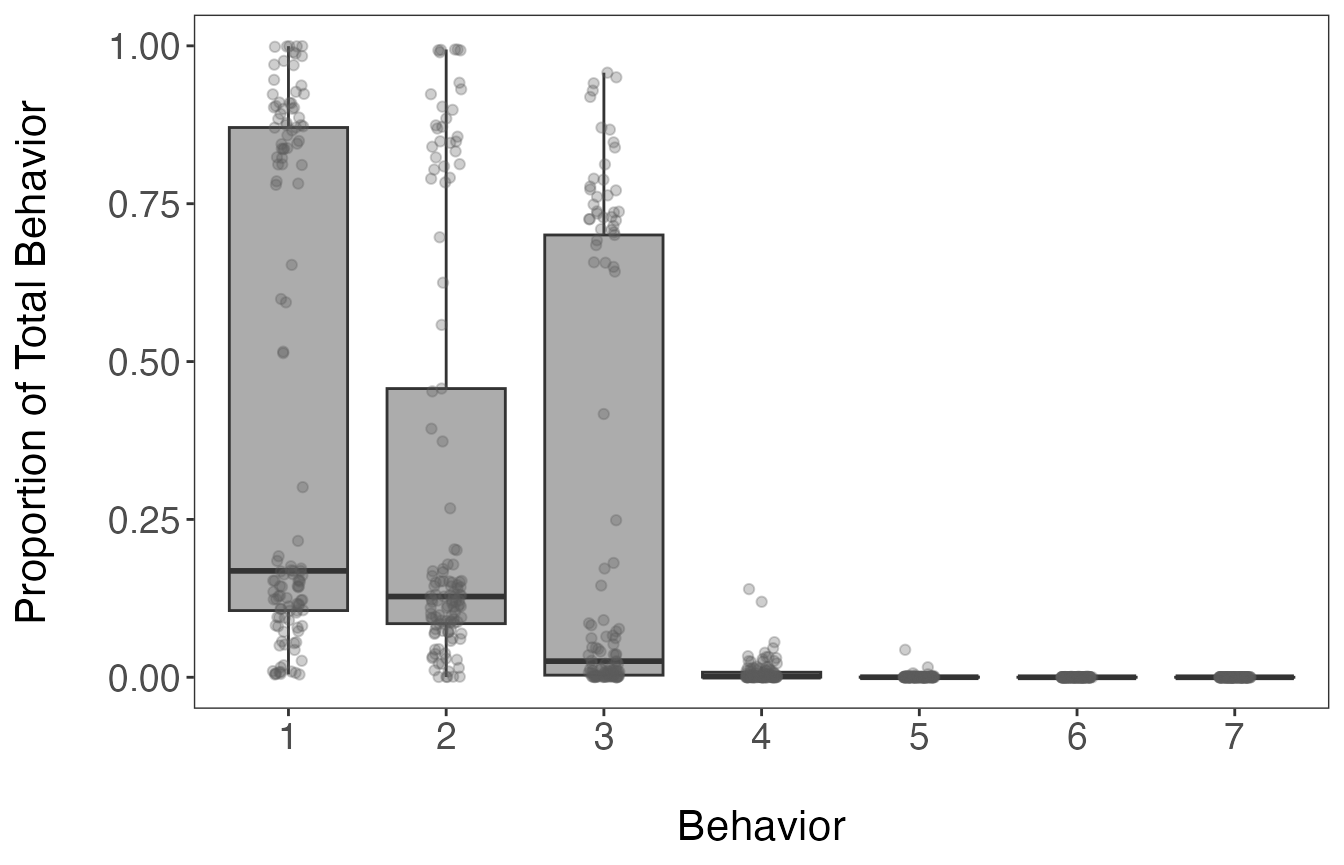The inspection of the mean proportions of behavioral states stored in the theta.estim object shows that the first 3 states are attributed to 99.1% of observations. All of the estimates per segment and behavioral state are shown in the above boxplot.

### Classify the states as behaviors

Now that it appears that 3 states are likely present in this dataset, we need to extract the state-dependent distributions from the $$\phi$$ matrix using the get_behav_hist() function. By visualizing these distributions, we can verify the findings determined using the $$\theta$$ matrix and assign names to the states based on the shape of the state-dependent distributions. Below, only the retained states have distributions shown in color. The remaining 4 states are included to demonstrate that they are not biologically interpretable by comparison.

# Extract bin estimates from phi matrix
behav.res<- get_behav_hist(dat = res, nburn = nburn, ngibbs = ngibbs, nmaxclust = nmaxclust,
var.names = c("Step Length","Turning Angle","Resting"))

# Plot histograms of proportion data
ggplot(behav.res, aes(x = bin, y = prop, fill = as.factor(behav))) +
geom_bar(stat = 'identity') +
labs(x = "\nBin", y = "Proportion\n") +
theme_bw() +
theme(axis.title = element_text(size = 16),
axis.text.y = element_text(size = 14),
axis.text.x.bottom = element_text(size = 12),
strip.text = element_text(size = 14),
strip.text.x = element_text(face = "bold")) +
scale_fill_manual(values = c("#21908CFF","#440154FF","#FDE725FF",
"grey35","grey35","grey35","grey35"), guide = "none") +
scale_y_continuous(breaks = c(0.00, 0.50, 1.00)) +
scale_x_continuous(breaks = 1:8) +
facet_grid(behav ~ var, scales = "free_x")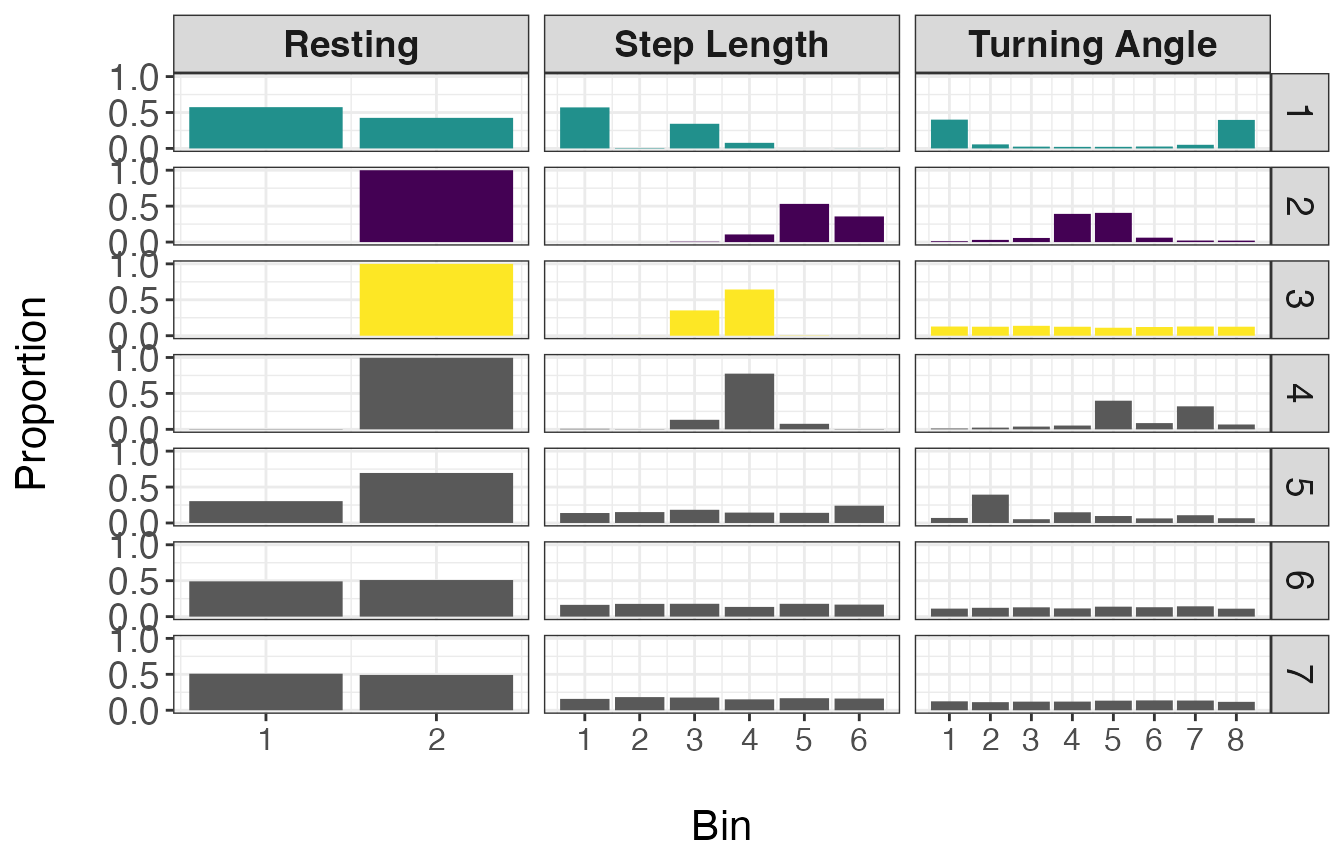From the above plot, it appears that state 1 has a high number of resting observations (bin 1 of Resting), short step lengths (primarily zero as exemplified by the high proportion in bin 1), and high turning angles (near $$\pi$$ or $$-\pi$$). Given these distributions, I will call state 1 ‘Resting’. States 2 and 3 have zero observations with resting behavior, but state 2 has larger step lengths and turning angles closer to zero, indicating relatively straight movements. Therefore state 2 will be called ‘Transit’ and state 3 will be called ‘Exploratory’.

Since each segment is defined as a mixture of the different behavioral states, we will need to assign these proportions back to our dataset. These proportions can also be visualized over time.

# Reformat proportion estimates for all track segments
theta.estim.long<- expand_behavior(dat = tracks.seg, theta.estim = theta.estim, obs = obs,
nbehav = 3, behav.names = c("Resting", "Transit", "Exploratory"),
behav.order = c(1,3,2))

# Plot results
ggplot(theta.estim.long) +
geom_area(aes(x=date, y=prop, fill = behavior), color = "black", size = 0.25,
position = "fill") +
labs(x = "\nTime", y = "Proportion of Behavior\n") +
scale_fill_viridis_d("Behavior") +
theme_bw() +
theme(axis.title = element_text(size = 16),
axis.text.y = element_text(size = 14),
axis.text.x.bottom = element_text(size = 12),
strip.text = element_text(size = 14, face = "bold"),
panel.grid = element_blank()) +
facet_wrap(~id, nrow = 3)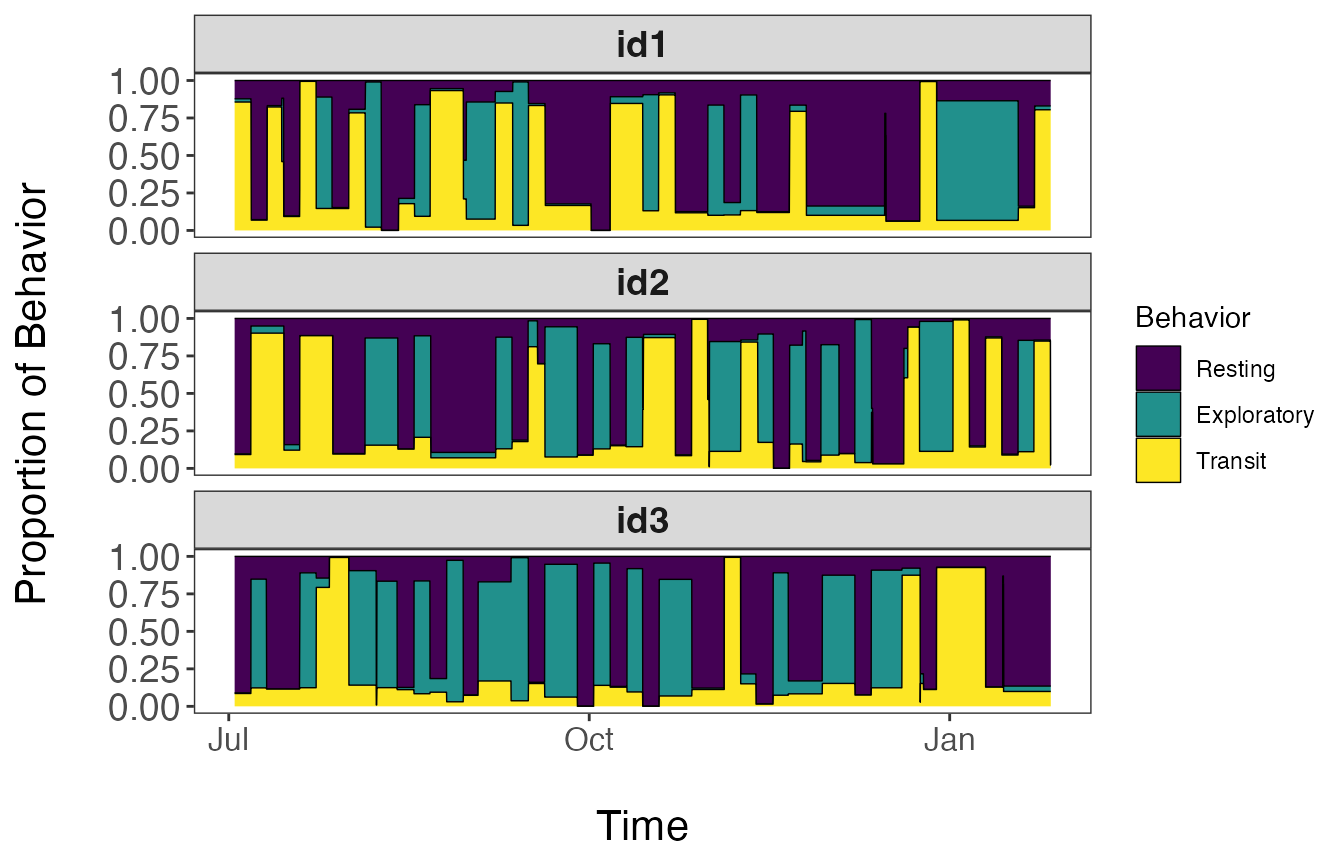## Assign behavioral states to tracks

The last major step is to now assign the behavioral states back to the original tracks. More specifically, the proportion of each behavioral state assigned to each track segment will be associated with all observations in that particular track segment. When mapping these results, users can either plot the dominant behavior per observation or potentially the proportion associated with a given behavioral state of interest.

# Merge results with original data
tracks.out<- assign_behavior(dat.orig = tracks,
dat.seg.list = df_to_list(tracks.seg, "id"),
theta.estim.long = theta.estim.long,
behav.names = c("Resting","Exploratory","Transit"))

# Map dominant behavior for all IDs
ggplot() +
geom_path(data = tracks.out, aes(x=x, y=y), color="gray60", size=0.25) +
geom_point(data = tracks.out, aes(x, y, fill=behav), size=1.5, pch=21, alpha=0.7) +
geom_point(data = tracks.out %>%
group_by(id) %>%
slice(which(row_number() == 1)) %>%
ungroup(), aes(x, y), color = "green", pch = 21, size = 3, stroke = 1.25) +
geom_point(data = tracks.out %>%
group_by(id) %>%
slice(which(row_number() == n())) %>%
ungroup(), aes(x, y), color = "red", pch = 24, size = 3, stroke = 1.25) +
scale_fill_viridis_d("Behavior", na.value = "grey50") +
labs(x = "Easting", y = "Northing") +
theme_bw() +
theme(axis.title = element_text(size = 16),
strip.text = element_text(size = 14, face = "bold"),
panel.grid = element_blank()) +
guides(fill = guide_legend(label.theme = element_text(size = 12),
title.theme = element_text(size = 14))) +
facet_wrap(~id, scales = "free", ncol = 2)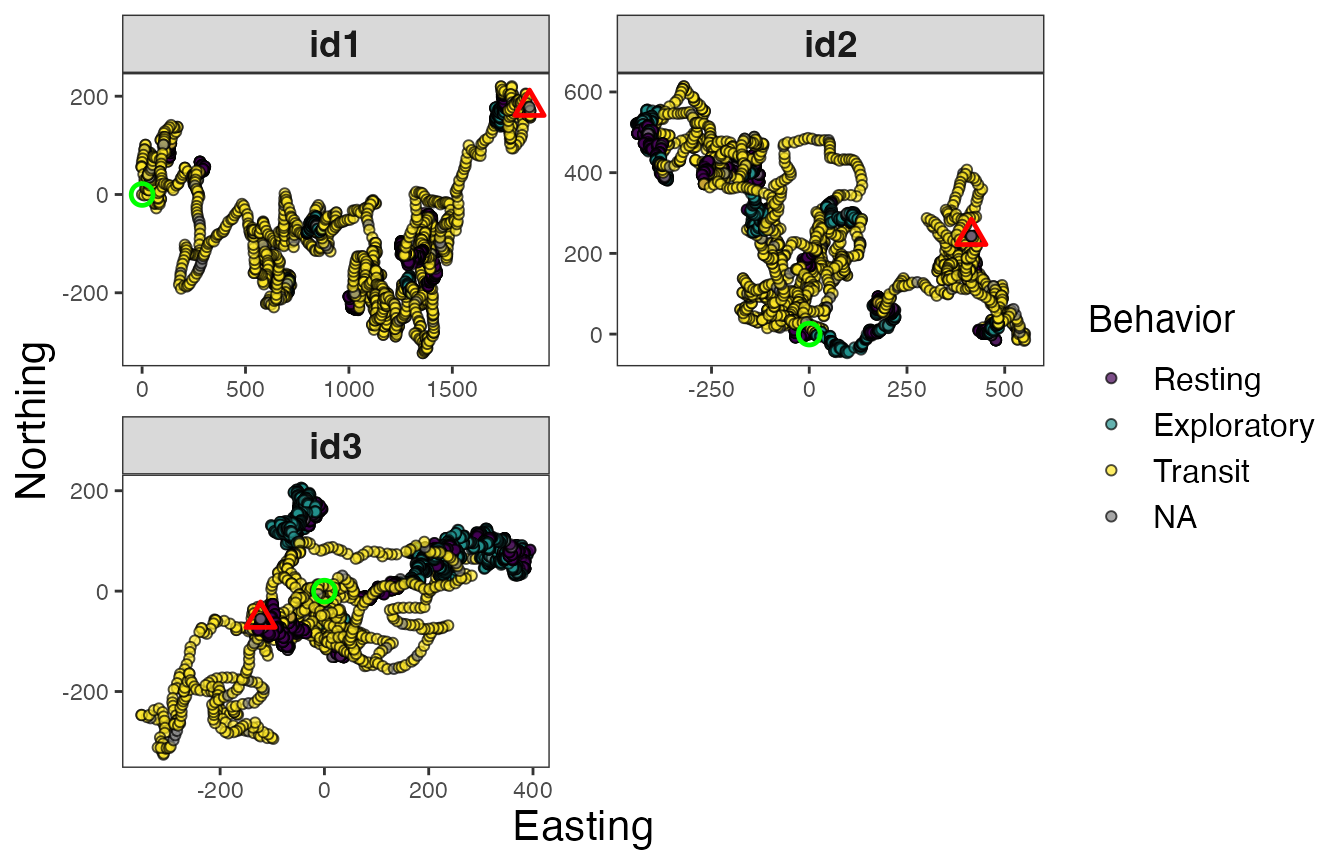# Map of all IDs
ggplot() +
geom_path(data = tracks.out, aes(x, y, color = behav, group = id), size=0.5) +
geom_point(data = tracks.out %>%
group_by(id) %>%
slice(which(row_number() == 1)) %>%
ungroup(), aes(x, y), color = "green", pch = 21, size = 3, stroke = 1.25) +
geom_point(data = tracks.out %>%
group_by(id) %>%
slice(which(row_number() == n())) %>%
ungroup(), aes(x, y), color = "red", pch = 24, size = 3, stroke = 1.25) +
scale_color_viridis_d("Behavior", na.value = "grey50") +
labs(x = "Easting", y = "Northing") +
theme_bw() +
theme(axis.title = element_text(size = 16),
strip.text = element_text(size = 14, face = "bold"),
legend.text = element_text(size = 12),
legend.title = element_text(size = 14))# Proportion resting
ggplot() +
geom_path(data = tracks.out, aes(x, y, color = Resting, group = id), size=0.5, alpha=0.7) +
geom_point(data = tracks.out %>%
slice(which(row_number() == 1)),
aes(x, y), color = "green", pch = 21, size = 3, stroke = 1.25) +
geom_point(data = tracks.out %>%
slice(which(row_number() == n())),
aes(x, y), color = "red", pch = 24, size = 3, stroke = 1.25) +
scale_color_distiller("Proportion\nResting", palette = "Spectral", na.value = "grey50") +
labs(x = "Easting", y = "Northing", title = "Resting") +
theme_bw() +
theme(axis.title = element_text(size = 16),
strip.text = element_text(size = 14, face = "bold"),
panel.grid = element_blank(),
legend.text = element_text(size = 12),
legend.title = element_text(size = 14))# Proportion exploratory
ggplot() +
geom_path(data = tracks.out, aes(x, y, color = Exploratory, group = id), size=0.5, alpha=0.7) +
geom_point(data = tracks.out %>%
slice(which(row_number() == 1)),
aes(x, y), color = "green", pch = 21, size = 3, stroke = 1.25) +
geom_point(data = tracks.out %>%
slice(which(row_number() == n())),
aes(x, y), color = "red", pch = 24, size = 3, stroke = 1.25) +
scale_color_distiller("Proportion\nExploratory", palette = "Spectral", na.value = "grey50") +
labs(x = "Easting", y = "Northing", title = "Exploratory") +
theme_bw() +
theme(axis.title = element_text(size = 16),
strip.text = element_text(size = 14, face = "bold"),
panel.grid = element_blank(),
legend.text = element_text(size = 12),
legend.title = element_text(size = 14))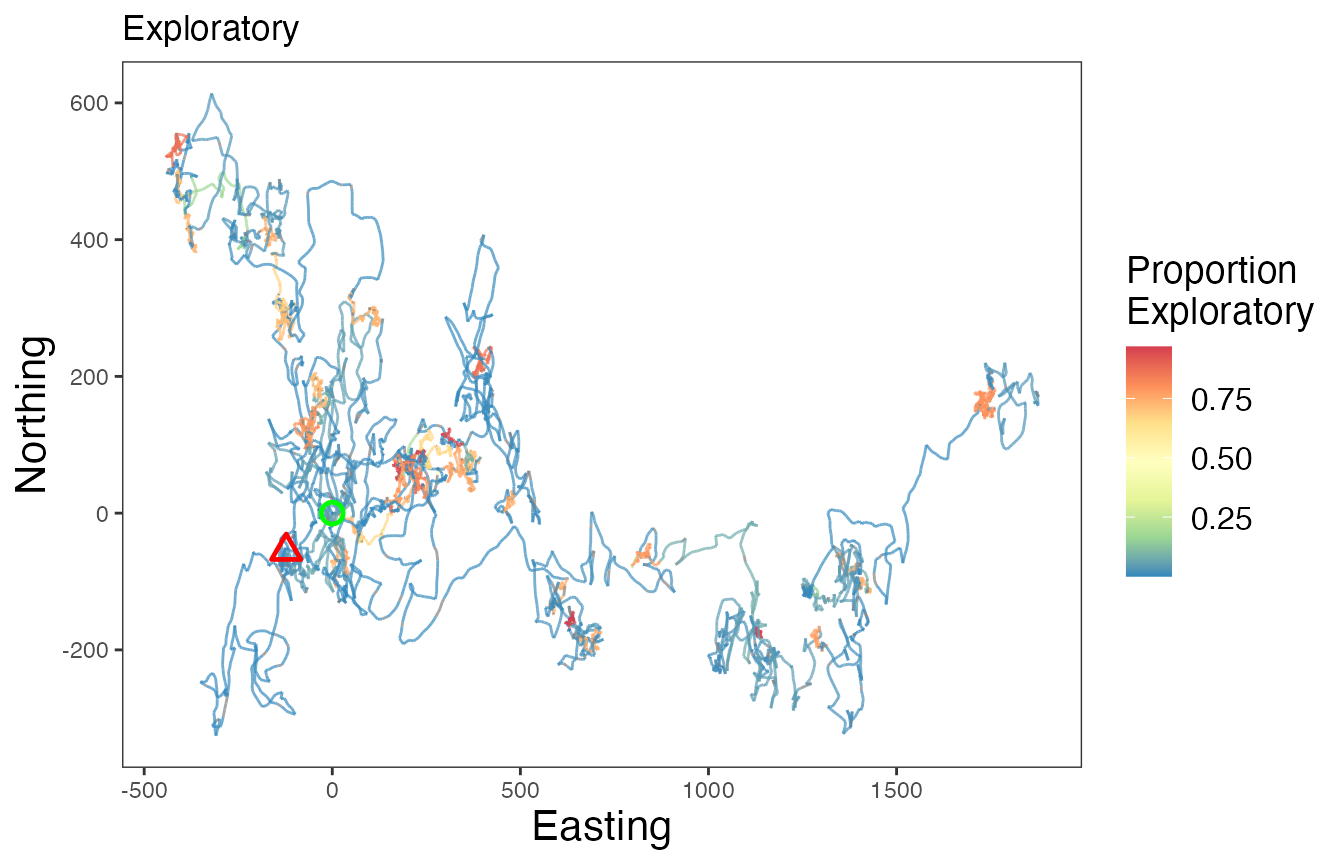# Proportion transit
ggplot() +
geom_path(data = tracks.out, aes(x, y, color = Transit, group = id), size=0.5, alpha=0.7) +
geom_point(data = tracks.out %>%
slice(which(row_number() == 1)),
aes(x, y), color = "green", pch = 21, size = 3, stroke = 1.25) +
geom_point(data = tracks.out %>%
slice(which(row_number() == n())),
aes(x, y), color = "red", pch = 24, size = 3, stroke = 1.25) +
scale_color_distiller("Proportion\nTransit", palette = "Spectral", na.value = "grey50") +
labs(x = "Easting", y = "Northing", title = "Transit") +
theme_bw() +
theme(axis.title = element_text(size = 16),
strip.text = element_text(size = 14, face = "bold"),
panel.grid = element_blank(),
legend.text = element_text(size = 12),
legend.title = element_text(size = 14))